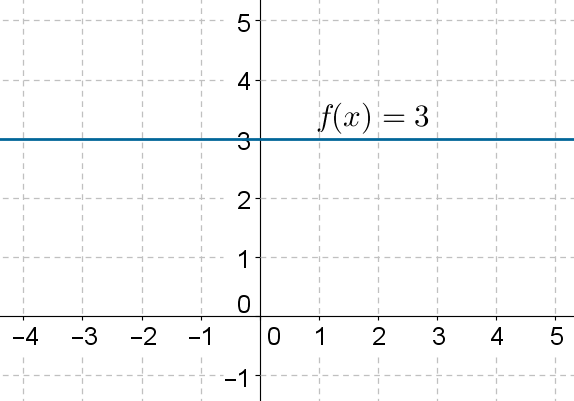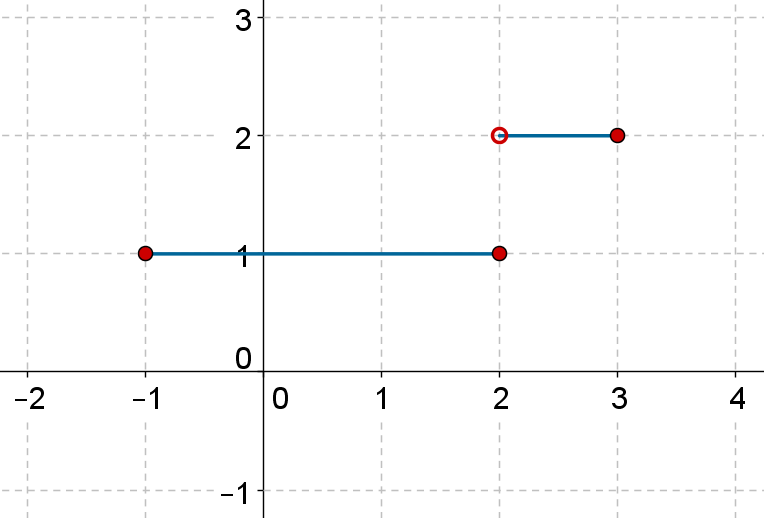# Constant Functions

Go back to  'Functions'

Consider the function

$f\left( x \right) = 3$

What does this definition mean? There is no variable in the definition (on the right side). This means that this particular function is a constant function, which means that it will always generate an output equal to 3, no matter what input value we give to it.

The graph of this function will be as follows:In general, a constant function will be of the form

$f\left( x \right) = k$

And the graph of this function will be a straight line parallel to the horizontal axis, passing through the point $$\left( {0,k}\right)$$.

Example 1: Plot the graph of

$f\left( x \right) = \left\{ \begin{array}{l}1, & x \in \left[ { - 1,2}\right]\\2, & x \in \left( {2,3} \right]\end{array} \right.$

Solution:The function has been defined piece wise, but it is constant in both parts(separately). The following is the graph of this function (note that solid dot at $$\left( {2,1} \right)$$ and the hollow dot at $$\left( {2,2} \right)$$; this indicates that $$f\left( 2 \right)$$equals 1 and not 2):Functions
Functions
grade 10 | Questions Set 2
Functions
Functions
grade 10 | Questions Set 1